Next: Plots Up: Z-plane, causality, and feedback Previous: Z-plane, causality, and feedback

# LEAKY INTEGRATION

The convolution equation ()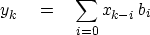(2)
says that the present output is created entirely from present and past values of the input. Now we will include past values of the output. The simplest example is numerical integration, such as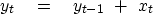(3)
Notice that when,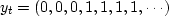,which shows that the integral of an impulse is a step.

A kind of deliberately imperfect integration used in numerical work is called leaky integration.''

The name derives from the analogous situation of electrical circuits, where the voltage on a capacitor is the integral of the current: in real life, some of the current leaks away. An equation to model leaky integration is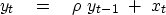(4)
whereis a constant that is slightly less than plus one. Notice that ifwere greater than unity, the output of (4) would grow with time instead of decaying. A program for this simple operation is leak(). I use this program so frequently that I wrote it so the output could be overlaid on the input. leak() uses a trivial subroutine, copy(), for copying.

subroutine leak( rho, n, xx, yy)
integer i, n;   real xx(n), yy(n), rho
temporary real tt( n)
call     null( tt, n)
tt(1) = xx(1)
do i= 2, n
tt(i) = rho * tt(i-1) + xx(i)
call copy( n, tt, yy)
return; end


Let us see what Z-transform equation is implied by (4). Move the y terms to the left:(5)
Given the Z-transform equation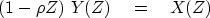(6)
notice that (5) can be derived from (6) by finding the coefficient of Zt. Thus we can say that the output Y(Z) is derived from the input X(Z) by the polynomial division(7)
Therefore, the effective filter B(Z) in Y(Z)=B(Z)X(Z) is(8)
The left side of Figure 1 shows a damped exponential function that consists of the coefficients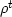seen in equation (8).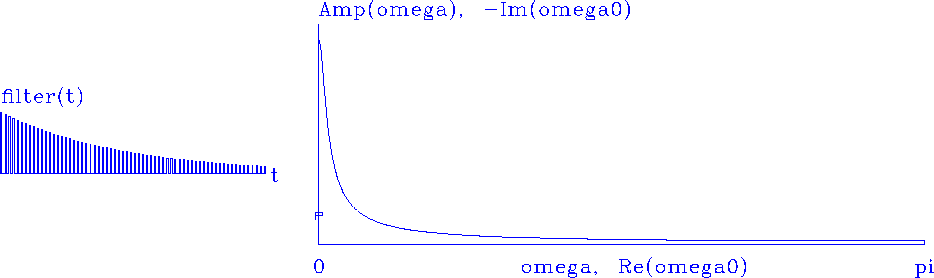leak
Figure 1
Left is the impulse response of leaky integration. Right is the amplitude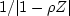in the Fourier domain.

The spectrum of bt is defined by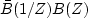.The amplitude spectrum is the square root of the spectrum.

It can be abbreviated by |B(Z)|. The amplitude spectrum is plotted on the right side of Figure 1. Ordinary integration has a Fourier responsethat blows up at.Leaky integration smooths off the infinite value at.Thus in the figure, the amplitude spectrum looks like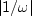,except that it is notat.Next: Plots Up: Z-plane, causality, and feedback Previous: Z-plane, causality, and feedback
Stanford Exploration Project
10/21/1998